# Calculate the oxidation number of sulphur, chromium and nitrogen in

Question:

Calculate the oxidation number of sulphur, chromium and nitrogen in $\mathrm{H}_{2} \mathrm{SO}_{5}, \mathrm{C}_{2} \mathrm{O}_{7}^{2-}$ and $\mathrm{NO}_{3}^{-}$-. Suggest structure of these compounds. Count for the fallacy.

Solution: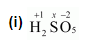$2(+1)+1(x)+5(-2)=0$

$\Rightarrow 2+x-10=0$

$\Rightarrow x=+8$

However, the O.N. of S cannot be +8. S has six valence electrons. Therefore, the O.N. of S cannot be more than +6.

The structure of $\mathrm{H}_{2} \mathrm{SO}_{5}$ is shown as follows: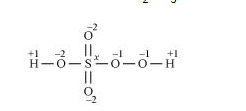Now, $2(+1)+1(x)+3(-2)+2(-1)=0$

$\Rightarrow 2+x-6-2=0$

$\Rightarrow x=+6$

Therefore, the $O \cdot N$. of $S$ is $+6$.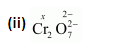$2(x)+7(-2)=-2$

$\Rightarrow 2 x-14=-2$

$\Rightarrow x=+6$

Here, there is no fallacy about the $\mathrm{O} . \mathrm{N}$. of $\mathrm{Cr}$ in $\mathrm{Cr}_{2} \mathrm{O}_{7}^{2-}$.

The structure of $\mathrm{Cr}_{2} \mathrm{O}_{7}^{2-}$ is shown as follows: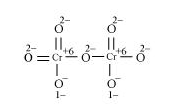Here, each of the two $\mathrm{Cr}$ atoms exhibits the O.N. of $+6$.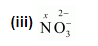$1(x)+3(-2)=-1$

$\Rightarrow x-6=-1$

$\Rightarrow x=+5$

Here, there is no fallacy about the $\mathrm{O} . \mathrm{N}$. of $\mathrm{N}$ in $\mathrm{NO}_{3}^{-}$.

The structure of $\mathrm{NO}_{3}^{-}$is shown as follows: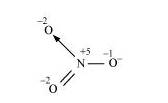The $N$ atom exhibits the $O \cdot N$. of $+5$.